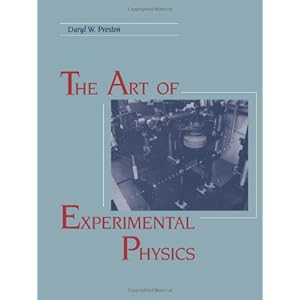This page was designed to give you the important and hard to find educational physics content which is a supplement to the other great educational physics materials on the web. Some recommendations include:

MIT Opencourseware Materials -http://ocw.mit.edu/courses/physics/
Wikipedia Physics Page

# Physics

We will begin this complete undergrad physics review "cheat sheet" with discussions of important or central concepts which we learn and prove experimentally in General Physics 1 and 2. Essentially everything they teach you in General Physics 1 and 2, as well as some further elaboration into the higher level course materials which you should find helpful and interesting.

## Classical Physics

### Kinematics & Newton's Laws of Motion

Kinematics is a study of the basic motions of objects. To understand the motions of an object and all the forces that object is experiencing all you really need to look at is position over time. Where the object is, and where it moves over time. From this we can mathematically infer all the forces the object is experiencing by using calculus to examine how that position changes as a function of time.

Acceleration: Forces are directly proportional to accelerations, which is a change in velocity over time (or change in position over time2). When you speed up or slow down, you are accelerating. "De-celeration" or slowing down, is the same as "negative acceleration" or acceleration in the opposite direction. The gas pedal in a car is sometimes called the "Accelerator" for this reason.

Velocity: Velocity is a change in position over time. Any time you change your velocity you are accelerating and thus experiencing unbalanced forces. Even if the forces are balanced that doesn't mean you are standing still though, it just means that your velocity isn't changing. That velocity could be a constant, like when you are travelling in a car at 70 Mph, you don't feel any outside forces unless the car turns or jams on the brakes. With a constant acceleration a over a time period t the velocity v=at, (for a changing acceleration we would need an integral sum over a*dt).

Position: We are left with the fundamental kinematics equation for position over time, take the initial position Xo and add the position as a function of initial velocity Xv=vt, and the position as a function of acceleration Xa=1/2at2 and we get Xa + Xv + Xo or 1/2at2 + vt + Xo = X , where X is the measured position of the object.

## Modern Physics

An Introduction to Quantum Mechanics and Relativity.

Suggested Reading: "Thirty years that shook physics" - The Story of Quantum Theory by George Gamow

## Experimental Physics (Labs)"The Art of Experimental Physics" - by Daryl Preston

Daryle Preston is a professor/researcher at Yale and is a personal friend of my undergrad physics adviser. His book pretty much has it all, although you may also want to check out a few of these other useful books."An Introduction to Error Analysis: The Study of Uncertainties in Physical Measurements"- by John R. Taylor

Experimental Physics Labs: Please, use these labs as a guide or reference for your own work, and do not copy them or plagiarize any of the materials within them. Thanks. If you have any other undergrad experimental physics labs you would like to add or share with the group please contact me by email.

Simple Pendulum Damped Pendulum RLC Circuits: High Pass, Low Pass, and Band Pass Filters Torsional Harmonic Oscillator Fourier Series Expansion (of a square wave) Pulsed NMR - Nuclear Magnetic Resonance (with Hydrogen atoms) Hyperfine Splitting of Rubidium

## Mechanics

"If you can't build it, you don't understand it." - Richard Feynman

Suggested Reading: "Classical Dynamics - of particles and systems - Thornton and Marion

Mechanics has been described as the first major step from General Physics 1 and 2 where we learned basic simplified models of mechanical systems, where for simplicity things like frictional forces and resistance were generally ignored. In mechanics we learn about more accurate models which account for added factors such as air resistance and drag forces.

These are the most important and most useful concepts you learn in Mechanics:
Hamilton's Principle
Lagrangian Mechanics
Gravitational Potential

## Electricity & Magnetism:I have no idea who came up with the joke to use Maxwell's Equations in combination with quotes from the book of Genesis about the creation of light, but it continues to be repeated. This one is good since it show both the integral (on the left) and differential forms (2 on right), of Maxwell's equations.Maxwell's Equations along with the continuity equation (in gold above), are sufficient for describing all measurable and observable phenomena related to electricity and magnetism.Maxwells equations can also be converted into a wave equation which shows the velocity of these waves to be equal to the speed of light. In order to get this form take the curl of the curl for either B or E (Ampere's Law or Faraday's Law) use the BAC CAB rule and back substitution and you will end up with the wave equation:## Thermal Physics

The mathematics used to model thermodynamic systems is almost purely statistical mechanics, or statistical physics due to the large numbers of particles and energy states involved. The models attempt to take into account every single possible future state of the system and, of course, more homogenized (dis-ordered) systems are more probable, we get the laws on increasing entropy, and a mathematical understanding of the underpinnings of thermodynamic principals.

## Quantum Physics

There is the philosophical approach to Quantum Mechanics which examines how and why everything works this way, and then there is the method my University professors pushed on us: "Shut up and Calculate!" Schrodinger Equation, Hilbert Spaces, just the straight math and how to use it to get real answers to quantum physics problems like particle in a box, or a 1D, 2D, or 3D infinite well. Or just getting the simplest atom Hydrogen right, and getting the spectral emissions and intensities.